# Search by Topic

Filter by: Content type:
Age range:
Challenge level:

### There are 52 results

Broad Topics > Angles, Polygons, and Geometrical Proof > Quadrilaterals### Fractions Rectangle

##### Age 11 to 14 Challenge Level:

The large rectangle is divided into a series of smaller quadrilaterals and triangles. Can you untangle what fractional part is represented by each of the ten numbered shapes?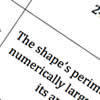### Shape Draw

##### Age 7 to 11 Challenge Level:

Use the information on these cards to draw the shape that is being described.### Poly Plug Rectangles

##### Age 5 to 14 Challenge Level:

The computer has made a rectangle and will tell you the number of spots it uses in total. Can you find out where the rectangle is?### Circles in Quadrilaterals

##### Age 14 to 16 Challenge Level:

Explore when it is possible to construct a circle which just touches all four sides of a quadrilateral.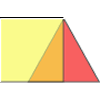### Overlaps

##### Age 5 to 7 Challenge Level:

What does the overlap of these two shapes look like? Try picturing it in your head and then use the interactivity to test your prediction.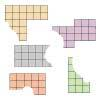### Torn Shapes

##### Age 7 to 11 Challenge Level:

These rectangles have been torn. How many squares did each one have inside it before it was ripped?### Trapezium Four

##### Age 14 to 16 Challenge Level:

The diagonals of a trapezium divide it into four parts. Can you create a trapezium where three of those parts are equal in area?### Areas of Parallelograms

##### Age 14 to 16 Challenge Level:

Can you find the area of a parallelogram defined by two vectors?### Property Chart

##### Age 11 to 14 Challenge Level:

A game in which players take it in turns to try to draw quadrilaterals (or triangles) with particular properties. Is it possible to fill the game grid?### Shapely Pairs

##### Age 11 to 14 Challenge Level:

A game in which players take it in turns to turn up two cards. If they can draw a triangle which satisfies both properties they win the pair of cards. And a few challenging questions to follow...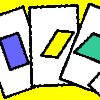### Quadrilaterals Game

##### Age 11 to 14 Challenge Level:

A game for 2 or more people, based on the traditional card game Rummy. Players aim to make two `tricks', where each trick has to consist of a picture of a shape, a name that describes that shape, and. . . .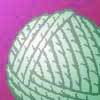### Stringy Quads

##### Age 7 to 11 Challenge Level:

This practical problem challenges you to make quadrilaterals with a loop of string. You'll need some friends to help!### Transformations on a Pegboard

##### Age 7 to 11 Challenge Level:

How would you move the bands on the pegboard to alter these shapes?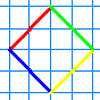### A Cartesian Puzzle

##### Age 7 to 11 Challenge Level:

Find the missing coordinates which will form these eight quadrilaterals. These coordinates themselves will then form a shape with rotational and line symmetry.### Rectangle Tangle

##### Age 7 to 11 Challenge Level:

The large rectangle is divided into a series of smaller quadrilaterals and triangles. Can you untangle what fractional part is represented by each of the shapes?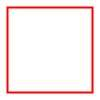### Numerically Equal

##### Age 7 to 11 Challenge Level:

Can you draw a square in which the perimeter is numerically equal to the area?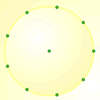### Quadrilaterals

##### Age 7 to 11 Challenge Level:

How many DIFFERENT quadrilaterals can be made by joining the dots on the 8-point circle?### Quad in Quad

##### Age 14 to 16 Challenge Level:

Join the midpoints of a quadrilateral to get a new quadrilateral. What is special about it?### Flexi Quads

##### Age 16 to 18 Challenge Level:

A quadrilateral changes shape with the edge lengths constant. Show the scalar product of the diagonals is constant. If the diagonals are perpendicular in one position are they always perpendicular?### Bendy Quad

##### Age 14 to 16 Challenge Level:

Four rods are hinged at their ends to form a convex quadrilateral. Investigate the different shapes that the quadrilateral can take. Be patient this problem may be slow to load.### Bracelets

##### Age 7 to 11 Challenge Level:

Investigate the different shaped bracelets you could make from 18 different spherical beads. How do they compare if you use 24 beads?### Triangle Transformation

##### Age 7 to 14 Challenge Level:

Start with a triangle. Can you cut it up to make a rectangle?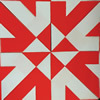### Folding Flowers 1

##### Age 7 to 11 Challenge Level:

Can you visualise what shape this piece of paper will make when it is folded?### An Equilateral Triangular Problem

##### Age 11 to 14 Challenge Level:

Take an equilateral triangle and cut it into smaller pieces. What can you do with them?### Completing Quadrilaterals

##### Age 11 to 14 Challenge Level:

We started drawing some quadrilaterals - can you complete them?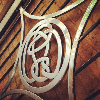### Guess My Quad

##### Age 11 to 14 Challenge Level:

How many questions do you need to identify my quadrilateral?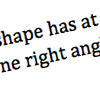### What Am I?

##### Age 7 to 11 Challenge Level:

Can you draw the shape that is being described by these cards?### Opposite Vertices

##### Age 11 to 14 Challenge Level:

Can you recreate squares and rhombuses if you are only given a side or a diagonal?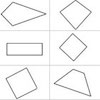### Quad Match

##### Age 7 to 11 Challenge Level:

A task which depends on members of the group noticing the needs of others and responding.### Square Pair

##### Age 16 to 18 Challenge Level:

Explore the shape of a square after it is transformed by the action of a matrix.### Bicentric Quadrilaterals

##### Age 14 to 16 Challenge Level:

Investigate the properties of quadrilaterals which can be drawn with a circle just touching each side and another circle just touching each vertex.### Cutting it Out

##### Age 5 to 11 Challenge Level:

I cut this square into two different shapes. What can you say about the relationship between them?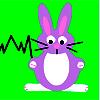### Rabbit Run

##### Age 7 to 11 Challenge Level:

Ahmed has some wooden planks to use for three sides of a rabbit run against the shed. What quadrilaterals would he be able to make with the planks of different lengths?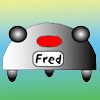### Fred the Class Robot

##### Age 7 to 11 Challenge Level:

Billy's class had a robot called Fred who could draw with chalk held underneath him. What shapes did the pupils make Fred draw?### Flexi Quad Tan

##### Age 16 to 18 Challenge Level:

As a quadrilateral Q is deformed (keeping the edge lengths constnt) the diagonals and the angle X between them change. Prove that the area of Q is proportional to tanX.### Folding

##### Age 7 to 11 Challenge Level:

What shapes can you make by folding an A4 piece of paper?### The Cyclic Quadrilateral

##### Age 11 to 16

This gives a short summary of the properties and theorems of cyclic quadrilaterals and links to some practical examples to be found elsewhere on the site.### Making Rectangles, Making Squares

##### Age 11 to 14 Challenge Level:

How many differently shaped rectangles can you build using these equilateral and isosceles triangles? Can you make a square?### Cyclic Quad Jigsaw

##### Age 14 to 16 Challenge Level:

A picture is made by joining five small quadrilaterals together to make a large quadrilateral. Is it possible to draw a similar picture if all the small quadrilaterals are cyclic?### Linkage

##### Age 11 to 14 Challenge Level:

Four rods, two of length a and two of length b, are linked to form a kite. The linkage is moveable so that the angles change. What is the maximum area of the kite?### Arrowhead

##### Age 14 to 16 Challenge Level:

The points P, Q, R and S are the midpoints of the edges of a non-convex quadrilateral.What do you notice about the quadrilateral PQRS and its area?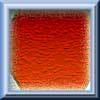### Hexagon Transformations

##### Age 7 to 11 Challenge Level:

Can you cut a regular hexagon into two pieces to make a parallelogram? Try cutting it into three pieces to make a rhombus!### Dividing the Field

##### Age 14 to 16 Challenge Level:

A farmer has a field which is the shape of a trapezium as illustrated below. To increase his profits he wishes to grow two different crops. To do this he would like to divide the field into two. . . .### Strange Rectangle 2

##### Age 16 to 18 Challenge Level:

Find the exact values of some trig. ratios from this rectangle in which a cyclic quadrilateral cuts off four right angled triangles.### Strange Rectangle

##### Age 16 to 18 Challenge Level:

ABCD is a rectangle and P, Q, R and S are moveable points on the edges dividing the edges in certain ratios. Strangely PQRS is always a cyclic quadrilateral and you can find the angles.### Long Short

##### Age 14 to 16 Challenge Level:

What can you say about the lengths of the sides of a quadrilateral whose vertices are on a unit circle?### Lawnmower

##### Age 14 to 16 Challenge Level:

A kite shaped lawn consists of an equilateral triangle ABC of side 130 feet and an isosceles triangle BCD in which BD and CD are of length 169 feet. A gardener has a motor mower which cuts strips of. . . .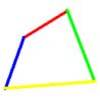### Biggest Bendy

##### Age 16 to 18 Challenge Level:

Four rods are hinged at their ends to form a quadrilateral. How can you maximise its area?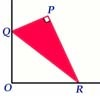### Set Square

##### Age 16 to 18 Challenge Level:

A triangle PQR, right angled at P, slides on a horizontal floor with Q and R in contact with perpendicular walls. What is the locus of P?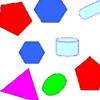### Part the Polygons

##### Age 7 to 11 Short Challenge Level:

Draw three straight lines to separate these shapes into four groups - each group must contain one of each shape.